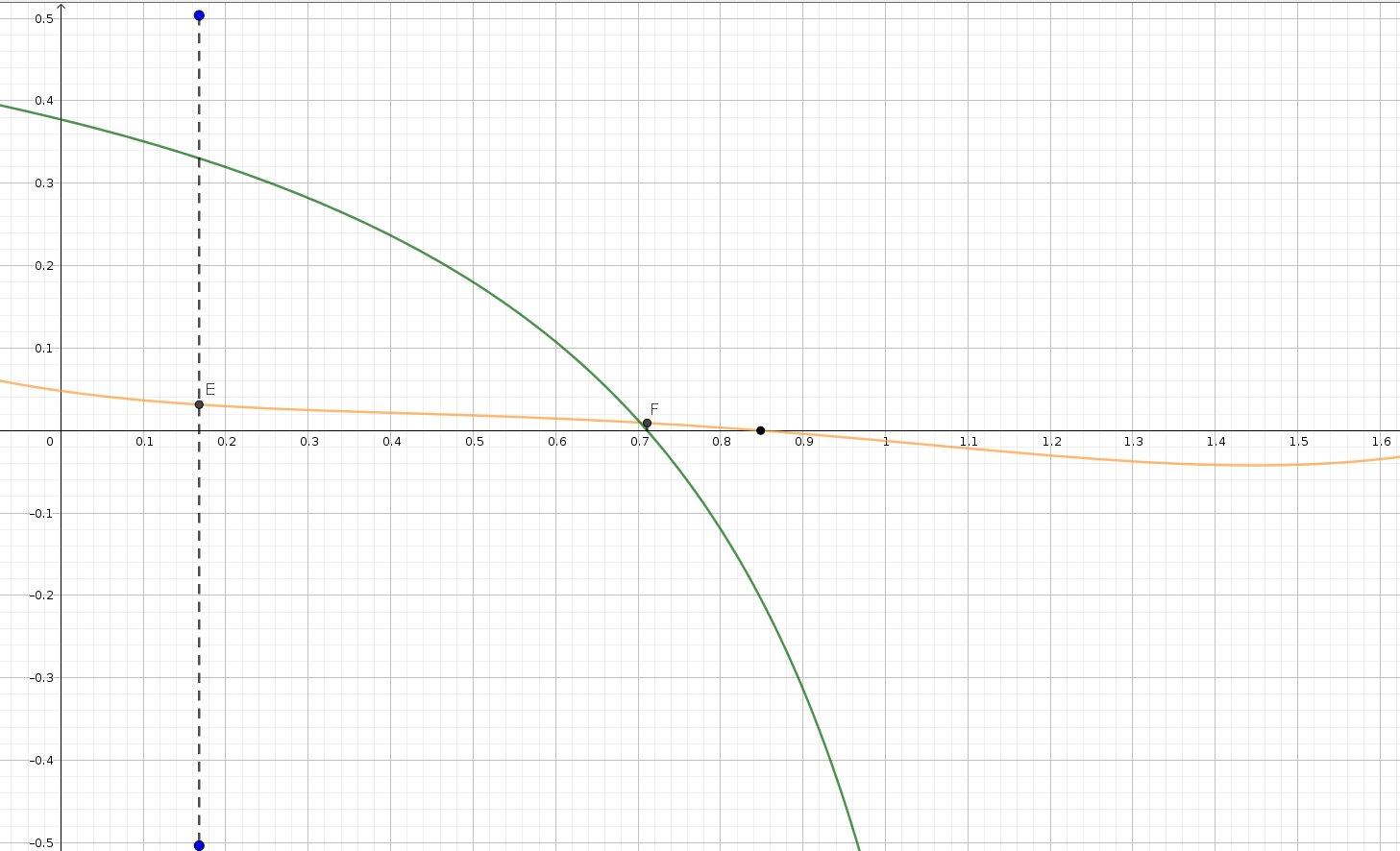#### Howdy, Stranger!

It looks like you're new here. Sign in or register to get started.

# Fitting cubic Bézier curves

2»

• I don't have a great answer to this question. In a hand-wavy sense, you're looking for answers that are "close" to the roots, and the real part is the closest you can get to the two complex roots.

I have a big pile of partially-finished code which you can find at https://github.com/raphlinus/raphlinus.github.io/tree/parallel_bezier, and an outline of a blog post at https://github.com/raphlinus/raphlinus.github.io/issues/80. Hopefully a real draft soon.
• edited September 2022
My question was not very precise. What I meant: Why do you consider complex roots at all? Looking at the Euler example, you would get with your method the x-coordinate of the point E and I would rather take the x-coordinate of F which has the lower y-coordinate than E and therefore is somehow "closer" to a root.• Right, I understand the question better. You definitely want to consider both E and F, and pick the one that is actually closer. There is no way to know a priori which is better, as that depends on the source curve. In this particular case, F is likely to be better. I didn't address that in the original blog post, but will in the followup. In any case, if you tweak the parameters a bit, then maybe E will dip closer to the x axis without actually crossing it, and then you definitely want to consider the complex root.

The d0=0 and d1=0 cases are boundary conditions. While keeping area exact, the moment goes through those cases smoothly, but the distance between curves (error metric) starts going up sharply when d is negative, and it's reasonable to reject those as unacceptable candidates without even measuring. (That's slightly less clear when doing offset curves, as you might be rendering a curve segment with a cusp, but I think it's valid even in that case).

So my current thinking is to throw those in as candidates, so you have up to six overall: four from the roots of the quartics, and d0=0 and d1=0. Then, as you point out, there's a potential issue that the tangent might not be accurate; in that case the tangent is determined by the second derivative as the first derivative is zero (L'Hospital). I'm going to look at handling those cases a bit differently - setting the other d to ensure the tangent (ie so the Bézier control point is at the intersection of the tangents) rather than the area, on the basis that tangents are more important.

(It might not be up to 6, though that's my current code. Rather, it might be better to consider these d=0 solutions when otherwise d would be negative.

The hypothesis is that evaluating these candidates is exhaustive, that there isn't a better fit somewhere else out there in parameter space. I don't have proof of this yet, but I'm starting to believe it's true based on experimentation. It's possible that random testing would help.
• I have done a test with 10⁷ random values for 0 < θ₀ < 90° and 0 < θ₁ < 90° and 0 < moment < 1 and 0 < area < 1:  There were plenty of cases where the real part of the complex roots were a better solution than the solutions I considered before. These cases were all for a small area and a big moment, which is probably unrealistic. When I changed to the condition 0.1 < area < 1, the real part of the complex roots was never the best solution. My test may contain errors. Therefore I would like to ask you if you know at least one realistic case, where the real part of the complex roots is the best case.
• Oh definitely, you see those cases quite prominently when curve-fitting Euler spirals, which was the first application domain I explored. I'm also seeing it in fitting parallel curves, which is what I've been working on the past few weeks.

I should also link my blog post, which has a bunch more detail on the refinements: https://raphlinus.github.io/curves/2022/09/09/parallel-beziers.html. That has live code as well. If you're handy with JS, it should be pretty easy to instrument that to print out the cases where the conjugate pair is the winning answer.